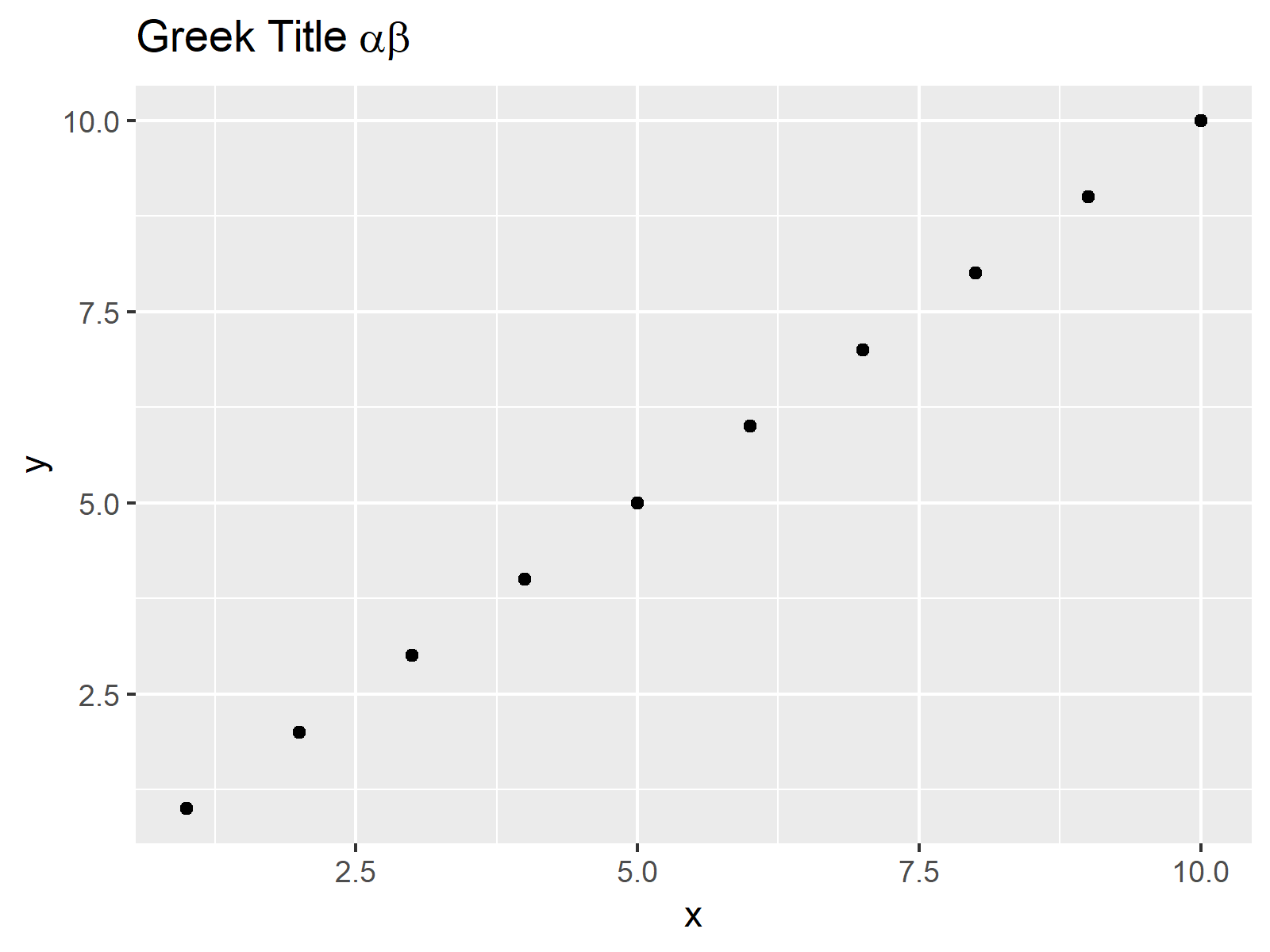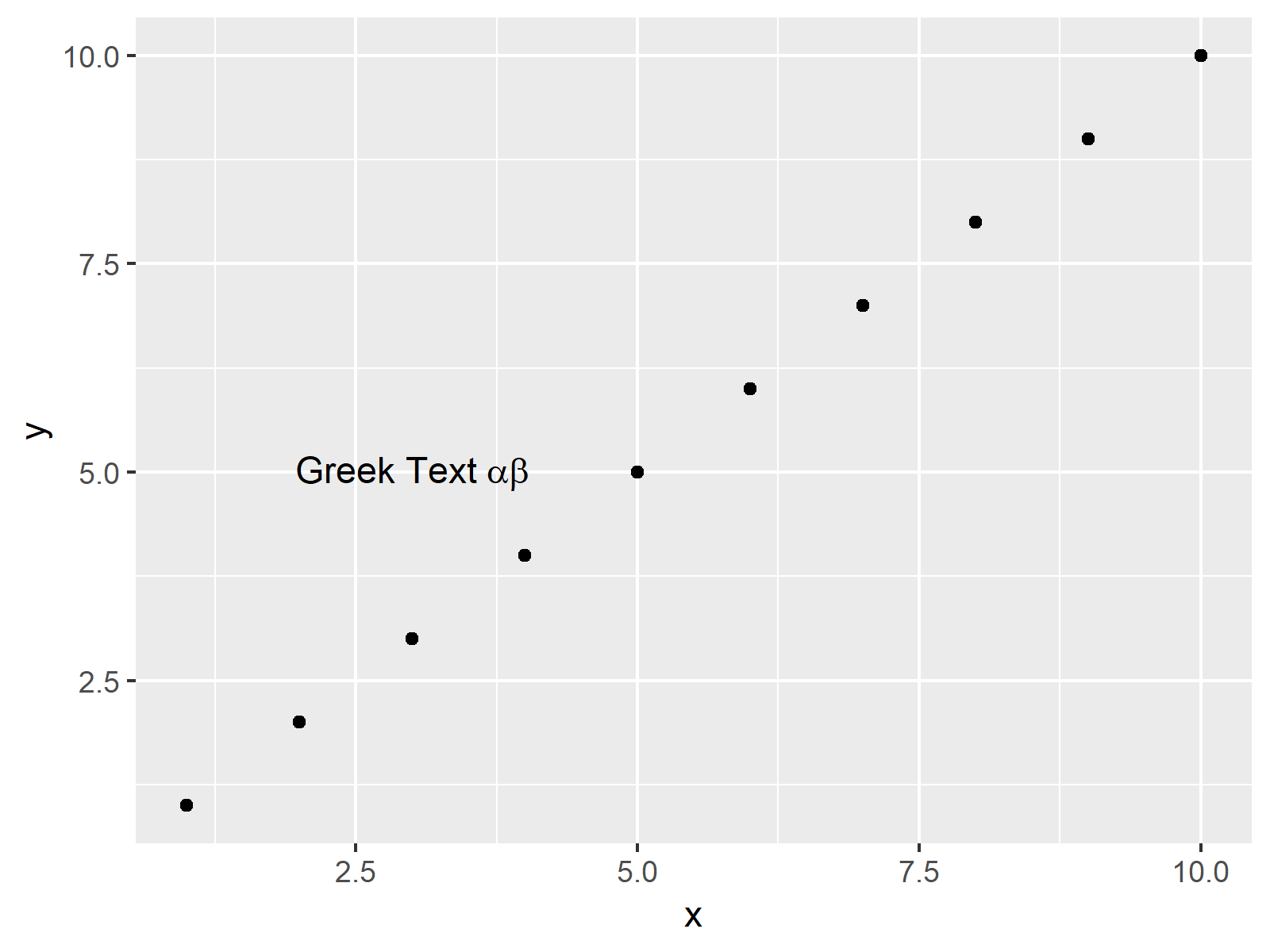# Add Greek Symbols to ggplot2 Plot in R (2 Examples)

In this tutorial you’ll learn how to create ggplot2 plots with Greek symbols in the R programming language.

The content of the article looks as follows:

Let’s dive right into the exemplifying R syntax:

## Introduction of Example Data

We’ll use the following data frame as example data in this R tutorial:

```data <- data.frame(x = 1:10,                            # Create example data
y = 1:10)```

Furthermore, we need to install and load the ggplot2 package to RStudio:

```install.packages("ggplot2")                             # Install and load ggplot2
library("ggplot2")```

Now, we can draw a ggplot2 graphic with the following R code:

```ggp <- ggplot(data, aes(x, y)) +                        # Create plot without text
geom_point()
ggp                                                     # Draw ggplot```Figure 1: ggplot2 Plot without Greek Symbols.

Figure 1 shows a basic ggplot2 scatterplot without any text elements. We’ll use this plot as basement for the following examples.

## Example 1: Adding Greek Symbols to Main Title

Example 1 explains how to add Greek symbols to a ggplot2 main title. In the following R syntax, we are using the expression function to draw the Greek letters alpha and beta:

```ggp +                                                   # Add Greek symbols to title
ggtitle(expression("Greek Title" ~ alpha * beta))```Figure 2: ggplot2 Plot with Greek Symbols in Main Title.

In Figure 2, you can see our updated plot including a main title with Greek symbols.

## Example 2: Adding Greek Symbols within Plot Area

We can also add Greek symbols within the plotting area of our graphic. For this task, we can use the annotate and the expression functions:

```ggp +                                                   # Add Greek symbols to plot
annotate("text", x = 3, y = 5,
label = expression("Greek Text" ~ alpha * beta))```Figure 3: ggplot2 Plot with Greek Symbols in Plot Area.

Figure 3 shows our ggplot2 graph with some text and Greek symbols in the middle of the plot.

## Video, Further Resources & Summary

Would you like to know more about drawing data with ggplot2? Then I can recommend to have a look at the following video of my YouTube channel. In the video, I explain the contents of this post in a live programming session.

In addition, you might have a look at the other R programming tutorials of my website. A selection of articles can be found below:

In this R tutorial you learned how to include Greek alphabet letters to a ggplot2 graphic. If you have additional questions or comments, please let me know in the comments.

Subscribe to the Statistics Globe Newsletter